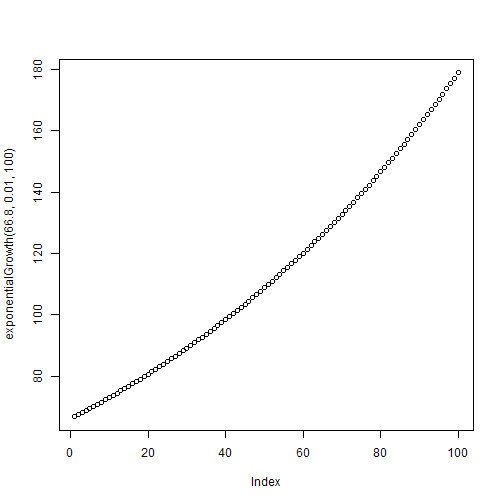``````x <- 1:5
sd(x)  # sd 是函数名, x 是自变量。
``````
``````##  1.581
``````

`sd()`用来计算标准差。当我们发出这条指令时，R 在幕后到底忙活个啥呢？输入函数名就能看到了：

``````sd
``````
``````## function (x, na.rm = FALSE)
## {
##     if (is.matrix(x)) {
##         msg <- "sd(<matrix>) is deprecated.\n Use apply(*, 2, sd) instead."
##         warning(paste(msg, collapse = ""), call. = FALSE, domain = NA)
##         apply(x, 2, sd, na.rm = na.rm)
##     }
##     else if (is.vector(x))
##         sqrt(var(x, na.rm = na.rm))
##     else if (is.data.frame(x)) {
##         msg <- "sd(<data.frame>) is deprecated.\n Use sapply(*, sd) instead."
##         warning(paste(msg, collapse = ""), call. = FALSE, domain = NA)
##         sapply(x, sd, na.rm = na.rm)
##     }
##     else sqrt(var(as.vector(x), na.rm = na.rm))
## }
## <bytecode: 0x0576f9bc>
## <environment: namespace:stats>
``````

``````assign("x", 1:5)
``````

``````newscore <- function(x) # newscore 是自定义的函数名，它有一个自变量 x。函数的返回值是 sqrt(x) * 10。
{
sqrt(x) * 10
}
``````

``````newscore(x = 40)
``````
``````##  63.25
``````

``````x <- 36
y <- 81
newscore(x = y)  # 函数内部的 x 把 81 的值传递进来，而不是36。
``````
``````##  90
``````

``````news <- function(x, n) {
sqrt(x) * 10 + n
}
news(x = 36, n = 10)
``````
``````##  70
``````
``````news(36, 10)  # 懒人为了省事儿，按自变量的默认顺序写就行了。
``````
``````##  70
``````
``````news(n = 10, x = 36)  # 如果打乱顺序，就必须指定谁是谁。
``````
``````##  70
``````

``````newscore()
``````
``````## Error: 'x' is missing
``````

``````newscore <- function(x = 36) # x 默认是 36。
{
sqrt(x) * 10
}
newscore()
``````
``````##  60
``````

``````exponentialGrowth <- function(N0, r = 0.01, tmax = 10) # 三个自变量：初始值，增长率，时间。
{
N <- N0
for (t in 1 : (tmax - 1)) {
N[t + 1] <- N[t] + r * N[t]
}
N # 这是最后一行，作为函数的返回值。
}

exponentialGrowth(66.8)
``````
``````##   66.80 67.47 68.14 68.82 69.51 70.21 70.91 71.62 72.33 73.06
``````
``````plot(exponentialGrowth(66.8, 0.01, 100))
````````````练习10.1 自定义一个名为 kaifang 的函数，用来开平方。

``````

``````length(unique(rownames(available.packages())))
``````

``````install.packages("maptools") # 第一次使用某个扩展包时要先安装。
``````
``````require(maptools)  # 调用扩展包，让 R 识别其中的函数。
``````
``````## Loading required package: maptools
``````
``````## Warning: package 'maptools' was built under R version 2.15.3
``````
``````## Loading required package: foreign
``````
``````## Loading required package: sp
``````
``````## Warning: package 'sp' was built under R version 2.15.3
``````
``````## Loading required package: grid
``````
``````## Loading required package: lattice
``````
``````## Checking rgeos availability: FALSE Note: when rgeos is not available,
## polygon geometry computations in maptools depend on gpclib, which has a
## restricted licence. It is disabled by default; to enable gpclib, type
## gpclibPermit()
``````
``````position <- c(116.39, 39.91)  # 旗杆的经纬度。
mydate <- "2013-03-24"  # 要计算的日期。
sunriset(matrix(position, nrow = 1), as.POSIXct(mydate, tz = "Asia/Shanghai"),
direction = c("sunrise"), POSIXct.out = TRUE)\$time  # 日出时间。
``````
``````##  "2013-03-24 06:11:53 CST"
``````
``````sunriset(matrix(position, nrow = 1), as.POSIXct(mydate, tz = "Asia/Shanghai"),
direction = c("sunset"), POSIXct.out = TRUE)\$time  # 日落时间。
``````
``````##  "2013-03-24 18:30:15 CST"
``````

``````flag <- function(date.start = "2013-03-24", date.length = 7) # 函数名为flag，默认是计算从前天起一周的升降器时刻。
{
mydate <- seq(as.POSIXct(date.start, tz="Asia/Shanghai"), by = 3600 * 24, length.out = date.length)
data.frame(sunrise = sunriset(matrix(c(116.39, 39.91), nrow = 1), as.POSIXct(mydate, tz="Asia/Shanghai"), direction=c("sunrise"), POSIXct.out = TRUE)\$time,
sunset = sunriset(matrix(c(116.39, 39.91), nrow = 1), as.POSIXct(mydate, tz="Asia/Shanghai"), direction=c("sunset"), POSIXct.out = TRUE)\$time)
}

flag("2013-10-01") # 好了，以后调用这个函数就能很方便计算。
``````
``````##               sunrise              sunset
## 1 2013-10-01 06:10:24 2013-10-01 17:57:17
## 2 2013-10-02 06:11:22 2013-10-02 17:55:40
## 3 2013-10-03 06:12:21 2013-10-03 17:54:03
## 4 2013-10-04 06:13:20 2013-10-04 17:52:27
## 5 2013-10-05 06:14:20 2013-10-05 17:50:51
## 6 2013-10-06 06:15:19 2013-10-06 17:49:16
## 7 2013-10-07 06:16:19 2013-10-07 17:47:41
``````

``````练习10.3 利用google earth 查出你所在地点的经纬度，然后利用 maptools 扩展包，计算你所在地点 2013 -2113 年 100 年的日出日落时间，然后通知记者来报导“退休职工某某某计算出某地日出日落时间表”。
``````

``````install.packages("animation")
require(animation)
demo("fireworks") # 会用网页浏览器打开一个动画。
citation("animation") # 看看作者。
``````

## 有用的信息：

( 连载中，待续 )

yangliufr 网友提了个好建议，希望给代码加上高亮。dapeng 试了一下，下面是个例子。但是 dapeng 懒得一一去改了，多包涵，凑合着看吧。

```flag <- function(date.start = "2013-03-24", date.length = 7) # 函数名为flag，默认是计算从前天起一周的升降器时刻。
{
mydate <- seq(as.POSIXct(date.start, tz="Asia/Shanghai"), by = 3600 * 24, length.out = date.length)
data.frame(sunrise = sunriset(matrix(c(116.39, 39.91), nrow = 1), as.POSIXct(mydate, tz="Asia/Shanghai"), direction=c("sunrise"), POSIXct.out = TRUE)\$time, sunset = sunriset(matrix(c(116.39, 39.91), nrow = 1), as.POSIXct(mydate, tz="Asia/Shanghai"), direction=c("sunset"), POSIXct.out = TRUE)\$time)
}
flag("2013-10-01") # 好了，以后调用这个函数就能很方便计算。
```

### 46 Responses to “R 菜鸟入门篇 第10篇 函数和包”

1. 这难度也太高了

• 动手试试，就不高了。

2. 果然趁热打铁，写的非常好啊。代码如果能像Rstudio里一样高亮，对于菜鸟来说，阅读起来可能更好一点。

• 谢谢鼓励。你的建议很好，以前我考虑过，只是懒得弄，被你这么一说，这回就试了试，用的是WP-Codebox插件，见正文末尾。
这个系列是用 Rsutdio 的 markdown 写成的，用 knitr 生成了 html 直接拷贝粘贴到博客里来，就成了这模样，原来 html 里的高亮都不见了，猜想可能是 wordpress 的 CSS 的设置导致的？这个我就外行了。

• 我用的Pretty R的网页代码生成工具，谷歌搜Pretty R就行。可以选择去掉他们的超链接。然后在编辑器里，点源代码编辑之后拷贝上去就好了。

• 好，试试你的主意：

```flag <- function(date.start = "2013-03-24", date.length = 7) # 函数名为flag，默认是计算从前天起一周的升降器时刻。
{
mydate <- seq(as.POSIXct(date.start, tz="Asia/Shanghai"), by = 3600 * 24, length.out = date.length)
data.frame(sunrise = sunriset(matrix(c(116.39, 39.91), nrow = 1), as.POSIXct(mydate, tz="Asia/Shanghai"), direction=c("sunrise"), POSIXct.out = TRUE)\$time, sunset = sunriset(matrix(c(116.39, 39.91), nrow = 1), as.POSIXct(mydate, tz="Asia/Shanghai"), direction=c("sunset"), POSIXct.out = TRUE)\$time)
}
flag("2013-10-01") # 好了，以后调用这个函数就能很方便计算。```

Created by Pretty R at inside-R.org

• 很不错！以后有集中的代码可以这么发布。只是这个方法对这个系列的帖子来说太麻烦了，懒得手动一段一段去改。

• 对 等以后完结了，想整个pdf的时候再弄

• 是的，如果有必要，我打算用 latex 的 listings 宏包来生成高亮代码的 pdf。

3. R现在有4365个包，哈哈，还可以更多啊

4. 农民工卡卡计算出驻马店1万年日出日落时间表。

小编们快来采访我吧！

5. 再一次膜拜谢老大

• 你是驻马店人？

6. dapeng的博文很生动哈，也比较由浅入深，每篇文章，我估计如果dapeng来讲课的话，恰好可以讲一节课，而且非常的有实操性。。。多谢dapeng 。

• 不客气。这个原本正是从课程讲义起源的啊！

7. 在调用library(tseries)时出现Error in library(tseries) : there is no package called ‘tseries’请问怎么解决 刚接触R谢谢你的博客

• 哈哈已经解决 可能这就是学习的乐趣

• 大概是没有成功安装或载入扩展包吧？解决问题的感觉的确很美好。

8. 请问楼主知道有木有月亮出没时间的package啊？小弟搜了一下下发觉没有个感觉

• 这个，好像真没有……不过有个包可以计算月球的位置，改一改应该可以算出月出月落的时刻。

9. 谢谢前阵子dapeng把最开始练习用的数据文件又特地上传了。今天抽空又补了几课。 没想到animation 的例子点开就是HAPPY NEW YEAR， 过年前还飘在国外自学软件的孩子看到了好惊喜！ 祝dapeng一家新年快乐。

• 哈哈，的确是巧了。新春快乐！

10. 博主，csv某一列的每个单元格里有多个分类变量，而且是用“；”隔开的。
如何把每个变量提取出来自成一行，其对应那一行的其他数据跟着重复？菜鸟还不会用HTML格式啊，见谅

• 老实说，我看不懂你的问题。给个例子吧。

• 额，这么说吧，csv第一列有三个数据(0.1)，(0.2),(0.3)。第二列分别对应(beijing)(shanghai;tianjing)，(zhenzhou;beijing;shenzhen)。我的问题之怎么用R软件将其变成第一列（0.1），（0.2），（0.2）（0.3）（0.3）（0.3），对应第二列（beijing）（shanghai）（tianjing）（zhenzhou）（beijing）（shehnzhen）

• 你的意思是，第一列是括号分隔的数据？0.2要重复2次，0.3要重复3次？依次跟六个城市对应？我觉得你还是直接把数据贴上来吧。多贴几行。

• columnA columnB
beijing 0.5454
beijing;shanghai 0.3536

11. columnA columnB
beijing 0.5454

12. 不是括号分割，是用的分号；确实根据第二列里的数据个数，重复第一列的内容

• 明白了。这个容易。先用字符串函数得到columnA里分号的数量n，然后让columnB重复n+1次，就可以对应上了，再生成个新列即可。你可以把数据发给我，我写个代码给你，一看就懂了。

• 请问怎么发给您？

• 联系方式

• 已发，博主好人

• 暂时还没收到。

• 额，不是dapengde(at)Live.com？

• 是，但你的邮件我没收到。

• 已再次发送

• 我把你的数据截取了一小段，放在了网上，方便代码读取和讨论，希望你不会介意。下面是代码。

``````data <- read.csv(file = 'http://pastebin.com/raw.php?i=vLL11Hr6', stringsAsFactors = FALSE)
newlist <- strsplit(data\$UCSC_RefGene_Group, ";")
newdata <- data.frame(unlist(newlist))
names(newdata) <- names(data)
comma_nr <- lapply(newlist, length)
data\$rep <- unlist(comma_nr)
newdata\$v <- rep(data\$X11N, data\$rep)
newdata
``````
• 非常感谢，数据用没有问题，以后可能还要多向您请教

• 不敢当。共同进步。

• LZ您好，首先感谢您的分享，很受用。针对“路今草”的问题，我写了一段
data

```m <- data.frame() for( i in 1:length(data\$columnB)) { strA <- toString(data\$columnA[i]) list <- unlist(strsplit(strA,";")) if(is.null(list)) { m <- cbind(strA,toString(data\$columnB[i])) } else { m <- cbind(list,(data\$columnB[i])) } } m ```

问题是会出现数据被覆盖的问题，它只显示最后两条，这是为什么

• ``````m <- cbind(list,(data\$columnB[i]))
``````

改成

``````m <- rbind(m, cbind(list,(data\$columnB[i])))
``````
13. sd这个内置函数的完整定义要怎么才能看到啊？

我在rstudio里面运行sd结果如下。说明文档里面也没有详细的定义

sd
function (x, na.rm = FALSE)
sqrt(var(if (is.vector(x)) x else as.double(x), na.rm = na.rm))

14. 很受用，我是你同学的学生，不知还有没有这种简单名了的资料。

• 据我所知，已经没有比这个更简单的了。